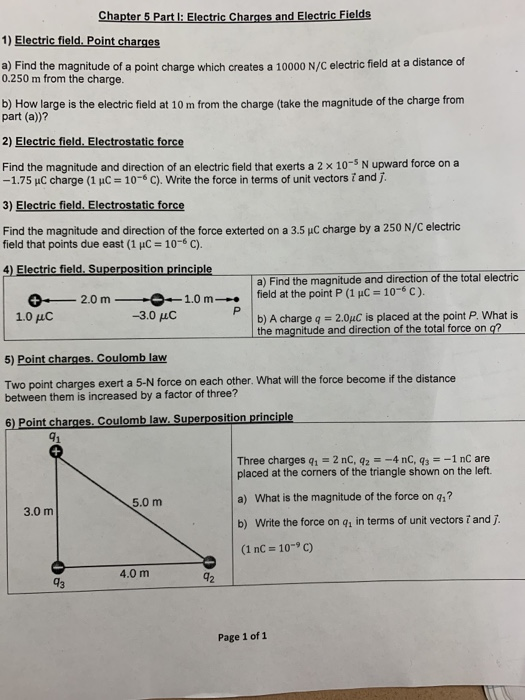# Question Solved1 AnswerChapter 5 Part I: Electric Charges and Electric Fields 1) Electric field. Point charges a) Find the magnitude of a point charge which creates a 10000 N/C electric field at a distance of 0.250 m from the charge. b) How large is the electric field at 10m from the charge (take the magnitude of the charge from part (a)? 2) Electric field. Electrostatic force Chapter 5 Part I: Electric Charges and Electric Fields 1) Electric field. Point charges a) Find the magnitude of a point charge which creates a 10000 N/C electric field at a distance of 0.250 m from the charge. b) How large is the electric field at 10m from the charge (take the magnitude of the charge from part (a)? 2) Electric field. Electrostatic force Find the magnitude and direction of an electric field that exerts a 2 x 10-5 N upward force on a -1.75 uC charge (1 uC 10- C). Write the force in terms of unit vectors 7 and j. 3) Electric field. Electrostatic force Find the magnitude and direction of the force exterted on a 3.5 uC charge by a 250 N/C electric field that points due east (1 uC 10- C). = 4) Electric field. Superposition principle a) Find the magnitude and direction of the total electric field at the point P (1 uC = 10-6 C). 1.0 m -3.0 μC 2.0 m P b) A charge q=2.0uC is placed at the point P What is the magnitude and direction of the total force on q? 1.0 μC 5) Point charges. Coulomb law Two point charges exert a 5-N force on each other. What will the force become if the distance between them is increased by a factor of three? 6) Point charges. Coulomb law. Superposition principle 91 Three charges q, = 2 nC, q2 =-4 nC, q3 =-1 nC are placed at the corners of the triangle shown on the left. a) What is the magnitude of the force on q,? 5.0 m 3.0 m b) Write the force on q, in terms of unit vectors 7 and (1 nC 10 C) 4.0 m 92 Page 1 of 1Transcribed Image Text: Chapter 5 Part I: Electric Charges and Electric Fields 1) Electric field. Point charges a) Find the magnitude of a point charge which creates a 10000 N/C electric field at a distance of 0.250 m from the charge. b) How large is the electric field at 10m from the charge (take the magnitude of the charge from part (a)? 2) Electric field. Electrostatic force Find the magnitude and direction of an electric field that exerts a 2 x 10-5 N upward force on a -1.75 uC charge (1 uC 10- C). Write the force in terms of unit vectors 7 and j. 3) Electric field. Electrostatic force Find the magnitude and direction of the force exterted on a 3.5 uC charge by a 250 N/C electric field that points due east (1 uC 10- C). = 4) Electric field. Superposition principle a) Find the magnitude and direction of the total electric field at the point P (1 uC = 10-6 C). 1.0 m -3.0 μC 2.0 m P b) A charge q=2.0uC is placed at the point P What is the magnitude and direction of the total force on q? 1.0 μC 5) Point charges. Coulomb law Two point charges exert a 5-N force on each other. What will the force become if the distance between them is increased by a factor of three? 6) Point charges. Coulomb law. Superposition principle 91 Three charges q, = 2 nC, q2 =-4 nC, q3 =-1 nC are placed at the corners of the triangle shown on the left. a) What is the magnitude of the force on q,? 5.0 m 3.0 m b) Write the force on q, in terms of unit vectors 7 and (1 nC 10 C) 4.0 m 92 Page 1 of 1
More
Transcribed Image Text: Chapter 5 Part I: Electric Charges and Electric Fields 1) Electric field. Point charges a) Find the magnitude of a point charge which creates a 10000 N/C electric field at a distance of 0.250 m from the charge. b) How large is the electric field at 10m from the charge (take the magnitude of the charge from part (a)? 2) Electric field. Electrostatic force Find the magnitude and direction of an electric field that exerts a 2 x 10-5 N upward force on a -1.75 uC charge (1 uC 10- C). Write the force in terms of unit vectors 7 and j. 3) Electric field. Electrostatic force Find the magnitude and direction of the force exterted on a 3.5 uC charge by a 250 N/C electric field that points due east (1 uC 10- C). = 4) Electric field. Superposition principle a) Find the magnitude and direction of the total electric field at the point P (1 uC = 10-6 C). 1.0 m -3.0 μC 2.0 m P b) A charge q=2.0uC is placed at the point P What is the magnitude and direction of the total force on q? 1.0 μC 5) Point charges. Coulomb law Two point charges exert a 5-N force on each other. What will the force become if the distance between them is increased by a factor of three? 6) Point charges. Coulomb law. Superposition principle 91 Three charges q, = 2 nC, q2 =-4 nC, q3 =-1 nC are placed at the corners of the triangle shown on the left. a) What is the magnitude of the force on q,? 5.0 m 3.0 m b) Write the force on q, in terms of unit vectors 7 and (1 nC 10 C) 4.0 m 92 Page 1 of 1